Next: Kirchhoff's Rules Up: Electric Current Previous: Emf and Internal Resistance

## Resistors in Series and in Parallel

Resistors are probably the most commonly occurring components in electronic circuits. Practical circuits often contain very complicated combinations of resistors. It is, therefore, useful to have a set of rules for finding the equivalent resistance of some general arrangement of resistors. It turns out that we can always find the equivalent resistance by repeated application of two simple rules. These rules relate to resistors connected in series and in parallel.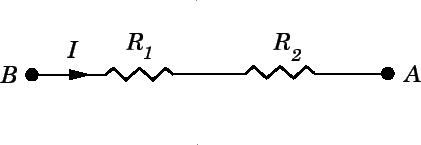Consider two resistors connected in series, as shown in Fig. 18. It is clear that the same currentflows through both resistors. For, if this were not the case, charge would build up in one or other of the resistors, which would not correspond to a steady-state situation (thus violating the fundamental assumption of this section). Suppose that the potential drop from pointto pointis. This drop is the sum of the potential dropsand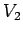across the two resistors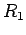and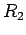, respectively. Thus,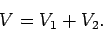(135)

According to Ohm's law, the equivalent resistance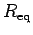betweenandis the ratio of the potential dropacross these points and the currentwhich flows between them. Thus,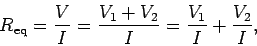(136)

giving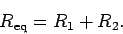(137)

Here, we have made use of the fact that the currentis common to all three resistors. Hence, the rule is
The equivalent resistance of two resistors connected in series is the sum of the individual resistances.
Forresistors connected in series, Eq. (137) generalizes to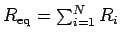.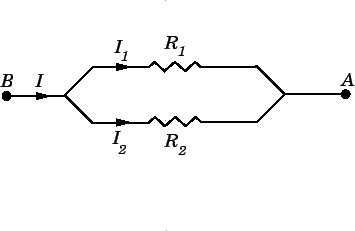Consider two resistors connected in parallel, as shown in Fig. 19. It is clear, from the figure, that the potential dropacross the two resistors is the same. In general, however, the currents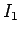and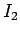which flow through resistorsand, respectively, are different. According to Ohm's law, the equivalent resistancebetweenandis the ratio of the potential dropacross these points and the currentwhich flows between them. This current must equal the sum of the currentsandflowing through the two resistors, otherwise charge would build up at one or both of the junctions in the circuit. Thus,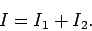(138)

It follows that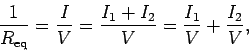(139)

giving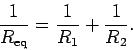(140)

Here, we have made use of the fact that the potential dropis common to all three resistors. Clearly, the rule is
The reciprocal of the equivalent resistance of two resistances connected in parallel is the sum of the reciprocals of the individual resistances.
Forresistors connected in parallel, Eq. (140) generalizes to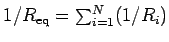.Next: Kirchhoff's Rules Up: Electric Current Previous: Emf and Internal Resistance
Richard Fitzpatrick 2007-07-14